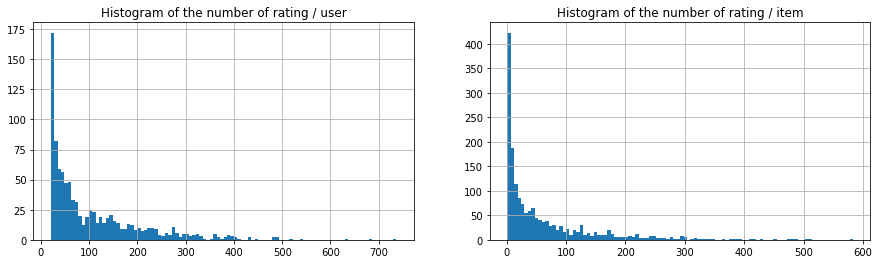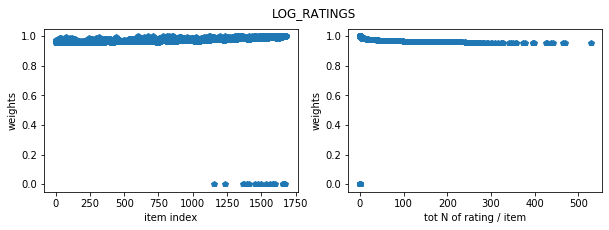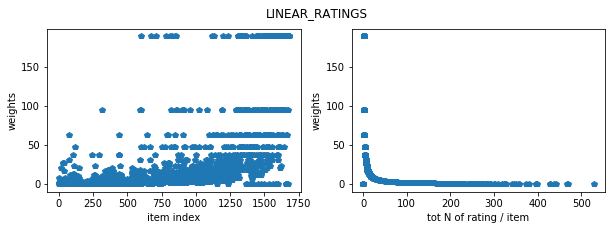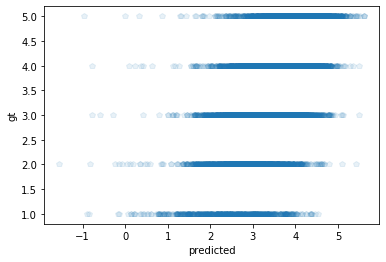# Learn about collaborative filtering and weighted alternating least square with tensorflow

In this blog, I will follow Recommendations in TensorFlow: Create the Model and study basic yet powerful recommendation algorithm, collaborative filtering using tensorflow version 1.

What you learn

• collaborative filtering
• Weighted alternating least squares (WALS) method
• tensorflow (v1.15.0)

In particular, this blog will show that the WALS method is pretty sensitive to the choice of weights (linear weights v.s. log weights vs uniform weights). I will use movieLens 100k data for demonstration. The data contains movie rating from 943 users for 1682 movies and the ratings range 1,2,...,5, with average rating of 3.5298. If you predicts all the testing ratings with this average rating value, the root mean squared error (RMSE) on testing data is 1.13. If we use the WALS method with various weighting schemes, after hyperparameter tunings, the optimized RMSE on testing data are:

weight type RMSE on training set RMSE on test set
baseline 1.12 1.13
uniform 2.35 2.51
linear 0.91 1.01
log 0.87 0.95
(The baseline method does not use collaborative filtering and predict all the ratings with average rating (3.5298)) Notice that the uniform method performs worse than the baseline, indicating that the choice of weights is extremely important.

# Reference¶

Recommendations in TensorFlow: Create the Model

In :
import warnings
warnings.filterwarnings('ignore')
import pandas as pd
import tensorflow as tf
import numpy as np
from scipy.sparse import coo_matrix
from tensorflow.contrib.factorization.python.ops import factorization_ops
print(tf.__version__)

1.15.0


First, publically available recommendation data: movielens.

curl -O 'http://files.grouplens.org/datasets/movielens/ml-1m.zip'
unzip ml-1m.zip
mkdir -p data
cp ml-1m/ratings.dat data/

Let's take a look at the data in my data/ folder:

In :
!ls
!echo ">>u.data"

data  ml-100k  ml-100k.zip  recommendation tensorflow.ipynb
>>u.data
196	242	3	881250949
186	302	3	891717742
22	377	1	878887116
244	51	2	880606923
166	346	1	886397596
298	474	4	884182806
115	265	2	881171488
253	465	5	891628467
305	451	3	886324817
6	86	3	883603013


## Data quick check¶

Data contains 100k ratings from 943 users. In total, there are 1682 items. You see 93% (1 - 100k/(1682*943)) of the (item, user) pairs are empty (i.e., not rated).The goal of the recommendation system problem here is to fill these empty cells.

In :
input_file = 'data/u.data'
headers = ['user_id', 'item_id', 'rating', 'timestamp']
sep='\t',
dtype={
'user_id': np.int32,
'item_id': np.int32,
'rating': np.float32,
'timestamp': np.int32,
})

def n_unique(colnm):
n = len(np.unique(ratings_df[colnm]))
return(n)
n_users = n_unique("user_id")
n_items = n_unique("item_id")
print("Total number of users:",n_users)
print("Total number of items:",n_items)

print("ratings_df.shape =",ratings_df.shape)

Total number of users: 943
Total number of items: 1682
ratings_df.shape = (100000, 4)

Out:
user_id item_id rating timestamp
0 196 242 3.0 881250949
1 186 302 3.0 891717742
2 22 377 1.0 878887116
3 244 51 2.0 880606923
4 166 346 1.0 886397596

The number of ratings per users and the number of ratings per items both have highly skewed distribution. Most of the users rate movies less than 100 times. Most of the items have less than 100 ratings. You will see later that the weights on the WALS algorithm essentiallily controls this skewed distribution by weighting each item (or user) differently, based on the frequency of the rating per item (per user).

In :
import matplotlib.pyplot as plt

fig, ax = plt.subplots(1,2,figsize=(15,4))
ratings_df.groupby("user_id").count()["item_id"].hist(bins=100,ax=ax)
_ = ax.set_title("Histogram of the number of rating / user")

ratings_df.groupby("item_id").count()["user_id"].hist(bins=100,ax=ax)
_ = ax.set_title("Histogram of the number of rating / item")The descriptive statistics show that item_id and user_id starts at 1.

In :
 ratings_df.describe()

Out:
user_id item_id rating timestamp
count 100000.00000 100000.000000 100000.000000 1.000000e+05
mean 462.48475 425.530130 3.529860 8.835289e+08
std 266.61442 330.798356 1.125674 5.343856e+06
min 1.00000 1.000000 1.000000 8.747247e+08
25% 254.00000 175.000000 3.000000 8.794487e+08
50% 447.00000 322.000000 4.000000 8.828269e+08
75% 682.00000 631.000000 4.000000 8.882600e+08
max 943.00000 1682.000000 5.000000 8.932866e+08

Normalize user_id and item_id so that the indexing starts at 0

In :
ratings = ratings_df[['user_id', 'item_id', 'rating']].values
ratings[:,0] -= 1
ratings[:,1] -= 1
print(ratings.shape,ratings.dtype)
for i in [0,1]:
print("start indexing at:",np.min(ratings[:,i]),"end indexint at:",np.max(ratings[:,i]))

(100000, 3) float64
start indexing at: 0.0 end indexint at: 942.0
start indexing at: 0.0 end indexint at: 1681.0


# Split between training and testing data¶

Here I select 10% of the data as test set. These ratings are removed from the training set and will be used to evaluate the predictive accuracy of the user and item factors. One note: This randomization is completely random. There is no stratification by users. So the same user may appears in both training adn testing. Notice that the sparse matrix dimension is the same between the training and testing.

The code is extracted from: _create sparse train and test

In :
def _create_sparse_train_and_test(ratings, n_users, n_items):
"""Given ratings, create sparse matrices for train and test sets.
Args:
ratings:  list of ratings tuples  (u, i, r)
n_users:  number of users
n_items:  number of items
Returns:
train, test sparse matrices in scipy coo_matrix format.

original extracted from:
"""
print(ratings.shape,ratings.min(),n_users,n_items)
# pick a random test set of entries, sorted ascending
test_set_size = int(len(ratings) * TEST_SET_RATIO)
np.random.seed(1)
test_set_idx = np.random.choice(range(len(ratings)),
size=test_set_size, replace=False)
test_set_idx = sorted(test_set_idx)

# sift ratings into train and test sets
ts_ratings = ratings[test_set_idx]
tr_ratings = np.delete(ratings, test_set_idx, axis=0)

# create training and test matrices as coo_matrix's
u_tr, i_tr, r_tr = zip(*tr_ratings)
tr_sparse = coo_matrix((r_tr, (u_tr, i_tr)), shape=(n_users, n_items))

u_ts, i_ts, r_ts = zip(*ts_ratings)
test_sparse = coo_matrix((r_ts, (u_ts, i_ts)), shape=(n_users, n_items))

return tr_sparse, test_sparse

## 10% of the data will be test set.
TEST_SET_RATIO = 0.1
tr_sparse, test_sparse = _create_sparse_train_and_test(ratings, n_users, n_items)

print("sparse matrix dimension:",tr_sparse.shape,tr_sparse.row.shape,tr_sparse.col.shape,tr_sparse.data.min())
print("sparse matrix dimension:",test_sparse.shape,test_sparse.row.shape,test_sparse.col.shape)

(100000, 3) 0.0 943 1682
sparse matrix dimension: (943, 1682) (90000,) (90000,) 1.0
sparse matrix dimension: (943, 1682) (10000,) (10000,)


## Weighted Alternating Least Square model¶

Let $n_r$ be the number of rows (users) and $n_c$ be the number of columns (items) in the observed sparse matrix $A$. In our case, $n_r=943$, $n_c=1682$ and, $A_{i,j}$ contains the $i$th user's rating on the $j$th movie (i.e, $A_{i,j}=1,2,...,5$). If there is no rating, $A_{i,j}=0$.

Let $p$ be number of latent_factor, in other words, the lower dimensional representation of the users and items. The WALS model minimizes the following loss with respect to $U \in R^{n_r \textrm{ x } p}$ and and $V^T \in R^{p \textrm{ x } n_c}$.

$$\|\sqrt W \odot (A - U V^T) \|_F^2 + \lambda (\|U\|_F^2 + \|V\|_F^2)$$

• $A \in R^{n_r \textrm{ x } n_c}$: observed sparse matrix, containing rating
• $U \in R^{n_r \textrm{ x } p }$: row factors, describing users
• $V \in R^{n_c \textrm{ x } p}$: column factors, describing items

Note that $\odot$ is Handmard product and it represents the element-wise multiplication between the weights $\sqrt{W}$ and the residual $(A - U V^T)$.

Once the matricies $U$ and $V$ are estimated, we can predict the rating of the $i$th user for the $j$th item as np.sum(U[i,:] * V[j,:]).

#### Weights¶

Each element of the weights are defined as:

\begin{array}{rll} W_{i,j}&=W_0 + R_i C_j&\textrm{if $A_{i,j}\ne 0$}\\ &=W_0 &\textrm{otherwise} \end{array}

• $W_0$: unobs_weight
• $R_i$: row_wts row weights
• $C_j$: col_wts column weights

## Uniform weights¶

If $W_0=0$ and $R_i=C_j=1$ then each observed rate (item, user) pair is treated equally. We call this default weight setting "uniform" weights. Since the number of ratings per item has highly skewed distribution, with uniform weights, some items that have substantially many ratings would dominate the row factors and column factors, which may not be ideal for predicting the rate on testing set in general.

## Log and linear weights $W$¶

You will find that the initialization of weights is extremely important in weighted alternating least squares (WALS) algorithm. The core function to define weights are make_wts.

Building a Recommendation System in TensorFlow: Overview briefly discusses linear and log weights for column weights. They are defined as:

### Linear weights¶

$$C_j = \frac{\texttt{feature_wt_factor}}{\textrm{N of users rating the jth item}}$$

### Log weights¶

$$C_j = \left(\frac{1}{\textrm{N of users rating the jth item}}\right)^{\texttt{feature_wt_exp}}$$ Notice that both weights are inversely related to the number of users rating the $j$th item, so that the weights down weights the item that have large number of observed ratings. The following code visualizes these weights for each item.

In :
def make_wts(data, wt_type, obs_wt, feature_wt_exp, axis):
"""Generate observed item weights.
Args:
data:             coo_matrix of ratings data
wt_type:          weight type, LOG_RATINGS or LINEAR_RATINGS
obs_wt:           linear weight factor
feature_wt_exp:   logarithmic weight factor
axis:             axis to make weights for, 1=rows/users, 0=cols/items
Returns:
vector of weights for cols (items) or rows (users)
"""
assert wt_type in ["LOG_RATINGS","LINEAR_RATINGS"]
# recipricol of sum of number of items across rows (if axis is 0)
frac = np.array(1.0/(data > 0.0).sum(axis))

# filter any invalid entries

# normalize weights according to assumed distribution of ratings
if wt_type == "LOG_RATINGS":
wts = np.array(np.power(frac, feature_wt_exp)).flatten()
elif wt_type == "LINEAR_RATINGS":
wts = np.array(obs_wt * frac).flatten()

# check again for any numerically unstable entries
assert np.isfinite(wts).sum() == wts.shape
return wts


### Visualization of weights¶

LINEAR RATINGS seems to put more weights on those items that are rated only a few times in comparisons to LOG RATINGS.

In :
n_nonzero_items = np.array((tr_sparse > 0).sum(axis=0)).squeeze()
print(n_nonzero_items.shape)

def plot(col_wt,n_nonzero_items,title):
fig, ax = plt.subplots(1,2,figsize=(10,3))
ax.plot(col_wt,"p")
ax.set_xlabel("item index")
ax.set_ylabel("weights")
ax.plot(n_nonzero_items,col_wt,"p")
ax.set_xlabel("tot N of rating / item")
ax.set_ylabel("weights")
plt.suptitle(title)
plt.show()

for wt_type in ["LOG_RATINGS","LINEAR_RATINGS"]:
col_wt = make_wts(tr_sparse, wt_type, obs_wt=189.8, feature_wt_exp=0.008, axis=0)
plot(col_wt,n_nonzero_items,title=wt_type)

(1682,)You may wonder why I set:

  obs_wt=189.8, feature_wt_exp=0.008

These are hyperparameters I take from Hyperparameter specification.

In :
def define_graph(data,PARAMS):
graph = tf.Graph()
with graph.as_default():

input_tensor = tf.SparseTensor(indices=np.array([data.row, data.col]).T,
values=(data.data).astype(np.float32),
dense_shape=data.shape)

row_wts = None
col_wts = None
num_rows = data.shape
num_cols = data.shape

# initialize the weights
if PARAMS["wt_type"] in ["LOG_RATINGS","LINEAR_RATINGS"]:
row_wts = np.ones(num_rows)
col_wts = make_wts(data,
PARAMS["wt_type"],
PARAMS['feature_wt_factor'],
PARAMS['feature_wt_exp'],axis=0)

model = factorization_ops.WALSModel(num_rows, num_cols, PARAMS["latent_factors"],
unobserved_weight=PARAMS["unobs_weight"],
regularization=PARAMS["regularization"],
row_weights=row_wts,
col_weights=col_wts)

return(graph,model,input_tensor)


# Training Functions¶

These functions are extracted from wals.py and modified to fit in this notebook.

In :
def train(graph,model,input_tensor,verbose=False):
sess = tf.Session(graph=graph)
with graph.as_default():
row_update_op = model.update_row_factors(sp_input=input_tensor)
col_update_op = model.update_col_factors(sp_input=input_tensor)

sess.run(model.initialize_op)
sess.run(model.worker_init)
for i in range(num_iterations):
sess.run(model.row_update_prep_gramian_op)
sess.run(model.initialize_row_update_op)
sess.run(row_update_op)
sess.run(model.col_update_prep_gramian_op)
sess.run(model.initialize_col_update_op)
sess.run(col_update_op)
if verbose and i % 1 == 0:
rf = sess.run(row_factor)
print("iter",i,rf.mean(),rf.min(),sess.run(col_factor).mean())
return sess

import math
def get_rmse(output_row, output_col, actual):
"""Compute rmse between predicted and actual ratings.
Args:
output_row: evaluated numpy array of row_factor
output_col: evaluated numpy array of col_factor
actual: coo_matrix of actual (test) values
Returns:
rmse
"""
mse = 0
rate_preds = []
for i in range(actual.data.shape):
row_pred = output_row[actual.row[i]]
col_pred = output_col[actual.col[i]]
rate_pred = np.dot(row_pred, col_pred)
rate_preds.append(rate_pred)
err = actual.data[i] - rate_pred
mse += err * err
mse /= actual.data.shape
rmse = math.sqrt(mse)
return rmse,rate_preds


# Hyperparameter tuning + training¶

I will set the following parameters fixed during the hyperparameter tunings. They are selected based on Hyperparameter specification.

In :
PARAMS = {
'regularization': 0.01,
'unobs_weight': .001,
'feature_wt_factor': 189.8,
'feature_wt_exp': 0.08,
}

In :
latent_factors  = [1,  2,   5, 15]
regularizations = [0.001,0.1,  5, 20,100]
wt_types = ["UNIFORM","LINEAR_RATINGS","LOG_RATINGS"]
rmse_best = np.Inf
num_iterations = 40
for wt_type in wt_types:
print(wt_type)
for lf in latent_factors:
for r in regularizations:
PARAMS["wt_type"] = wt_type
PARAMS["latent_factors"] = lf
PARAMS["regularizations"] = r

graph,model,input_tensor = define_graph(tr_sparse,PARAMS)
sess = train(graph,model,input_tensor)

output_row = model.row_factors.eval(session=sess)
output_col = model.col_factors.eval(session=sess)

rmse_train, _ = get_rmse(output_row, output_col, tr_sparse)
rmse_test, rate_preds_test = get_rmse(output_row, output_col, test_sparse)
print("{:10} latent factor {:3.0f}, reg {:5.1f}, rmse (train) {:5.2f}, rmse (test) {:5.2f}".format(" ",
lf,
r,
rmse_train,
rmse_test))
if rmse_test < rmse_best:
rmse_best = rmse_test
out = {
"rate_preds_test":rate_preds_test,
"rmse":rmse_test,
"output_row":output_row,
"output_col":output_col,
"params": PARAMS
}


UNIFORM
latent factor   1, reg   0.0, rmse (train)  2.87, rmse (test)  2.88
latent factor   1, reg   0.1, rmse (train)  2.87, rmse (test)  2.89
latent factor   1, reg   5.0, rmse (train)  2.87, rmse (test)  2.89
latent factor   1, reg  20.0, rmse (train)  2.87, rmse (test)  2.88
latent factor   1, reg 100.0, rmse (train)  2.87, rmse (test)  2.89
latent factor   2, reg   0.0, rmse (train)  2.75, rmse (test)  2.78
latent factor   2, reg   0.1, rmse (train)  2.75, rmse (test)  2.78
latent factor   2, reg   5.0, rmse (train)  2.75, rmse (test)  2.78
latent factor   2, reg  20.0, rmse (train)  2.75, rmse (test)  2.78
latent factor   2, reg 100.0, rmse (train)  2.75, rmse (test)  2.78
latent factor   5, reg   0.0, rmse (train)  2.57, rmse (test)  2.62
latent factor   5, reg   0.1, rmse (train)  2.57, rmse (test)  2.62
latent factor   5, reg   5.0, rmse (train)  2.57, rmse (test)  2.61
latent factor   5, reg  20.0, rmse (train)  2.57, rmse (test)  2.62
latent factor   5, reg 100.0, rmse (train)  2.57, rmse (test)  2.62
latent factor  15, reg   0.0, rmse (train)  2.36, rmse (test)  2.51
latent factor  15, reg   0.1, rmse (train)  2.35, rmse (test)  2.52
latent factor  15, reg   5.0, rmse (train)  2.35, rmse (test)  2.52
latent factor  15, reg  20.0, rmse (train)  2.36, rmse (test)  2.51
latent factor  15, reg 100.0, rmse (train)  2.35, rmse (test)  2.52
LINEAR_RATINGS
latent factor   1, reg   0.0, rmse (train)  1.15, rmse (test)  1.20
latent factor   1, reg   0.1, rmse (train)  1.22, rmse (test)  1.34
latent factor   1, reg   5.0, rmse (train)  1.24, rmse (test)  1.49
latent factor   1, reg  20.0, rmse (train)  1.24, rmse (test)  1.37
latent factor   1, reg 100.0, rmse (train)  1.24, rmse (test)  1.40
latent factor   2, reg   0.0, rmse (train)  0.95, rmse (test)  1.06
latent factor   2, reg   0.1, rmse (train)  0.91, rmse (test)  1.03
latent factor   2, reg   5.0, rmse (train)  0.90, rmse (test)  1.00
latent factor   2, reg  20.0, rmse (train)  0.92, rmse (test)  1.11
latent factor   2, reg 100.0, rmse (train)  0.90, rmse (test)  1.01
latent factor   5, reg   0.0, rmse (train)  0.82, rmse (test)  1.10
latent factor   5, reg   0.1, rmse (train)  0.82, rmse (test)  1.09
latent factor   5, reg   5.0, rmse (train)  0.81, rmse (test)  1.07
latent factor   5, reg  20.0, rmse (train)  0.81, rmse (test)  1.05
latent factor   5, reg 100.0, rmse (train)  0.81, rmse (test)  1.06
latent factor  15, reg   0.0, rmse (train)  0.64, rmse (test)  1.29
latent factor  15, reg   0.1, rmse (train)  0.63, rmse (test)  1.30
latent factor  15, reg   5.0, rmse (train)  0.64, rmse (test)  1.32
latent factor  15, reg  20.0, rmse (train)  0.64, rmse (test)  1.31
latent factor  15, reg 100.0, rmse (train)  0.64, rmse (test)  1.32
LOG_RATINGS
latent factor   1, reg   0.0, rmse (train)  0.93, rmse (test)  0.96
latent factor   1, reg   0.1, rmse (train)  0.93, rmse (test)  0.96
latent factor   1, reg   5.0, rmse (train)  0.93, rmse (test)  0.96
latent factor   1, reg  20.0, rmse (train)  0.93, rmse (test)  0.96
latent factor   1, reg 100.0, rmse (train)  0.93, rmse (test)  0.96
latent factor   2, reg   0.0, rmse (train)  0.87, rmse (test)  0.95
latent factor   2, reg   0.1, rmse (train)  0.88, rmse (test)  0.95
latent factor   2, reg   5.0, rmse (train)  0.87, rmse (test)  0.95
latent factor   2, reg  20.0, rmse (train)  0.87, rmse (test)  0.95
latent factor   2, reg 100.0, rmse (train)  0.87, rmse (test)  0.95
latent factor   5, reg   0.0, rmse (train)  0.80, rmse (test)  0.99
latent factor   5, reg   0.1, rmse (train)  0.80, rmse (test)  0.99
latent factor   5, reg   5.0, rmse (train)  0.80, rmse (test)  0.99
latent factor   5, reg  20.0, rmse (train)  0.80, rmse (test)  0.98
latent factor   5, reg 100.0, rmse (train)  0.80, rmse (test)  0.99
latent factor  15, reg   0.0, rmse (train)  0.60, rmse (test)  1.21
latent factor  15, reg   0.1, rmse (train)  0.60, rmse (test)  1.20
latent factor  15, reg   5.0, rmse (train)  0.60, rmse (test)  1.20
latent factor  15, reg  20.0, rmse (train)  0.60, rmse (test)  1.20
latent factor  15, reg 100.0, rmse (train)  0.60, rmse (test)  1.21


### Findings¶

• When the latent factor increases, the trainning rmse decreases but not the testing rmse.
• With uniform weights, the testing rmse is worse than guessing the rating with average rating.
• The log weighting performs the best in terms of testing rmse: 0.93

## Best model preference visualization on test data¶

The parameter configuation of the best hyperparameter combination is:

In :
out["params"]

Out:
{'regularization': 0.01,
'unobs_weight': 0.001,
'feature_wt_factor': 189.8,
'feature_wt_exp': 0.08,
'wt_type': 'LOG_RATINGS',
'latent_factors': 15,
'regularizations': 100}
In :
plt.plot(out["rate_preds_test"],test_sparse.data,"p",alpha=0.1)
plt.xlabel("predicted")
plt.ylabel("gt")
plt.show()